Equable Spherical Segment

EQUABLE SPHERICAL SEGMENT

Balmoral Software

Solutions: 0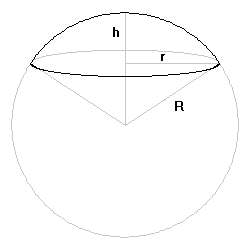Let R be the radius of a sphere and h the height of a spherical segment of one base, where 0 < h < 2R. The volume of the spherical segment is V, where
V/π = h2(R - h/3)
The lateral surface area of the spherical segment is Sl, where
Sl/π = 2Rh
The surface area of the base is Sb, where
Sb/π = r2
and r is the radius of the base. The above three quantities are integers by convention. The base radius is related to the sphere radius and segment height by
r2 = R2 - |R - h|2,
soIt follows that h2 is an integer and therefore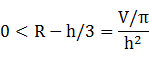is rational. Then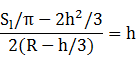is also rational. The fraction on the right is a quotient of two rational numbers, so 2h is rational and it follows that h is an integer.

The equability requirement is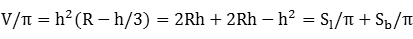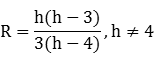Since h < 2R, we have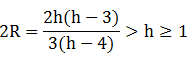4 < h < 6,

so h = 5. It follows from  that R = 10/3. But then Sb/π = 25/3 is not an integer, so there are no equable spherical segments with integer volume and surface area sections.